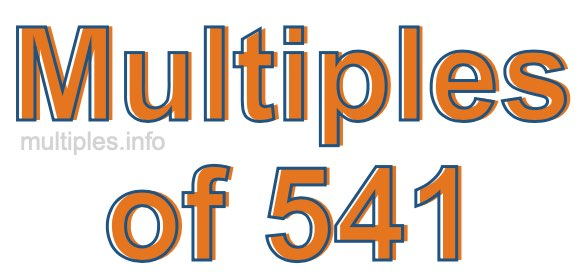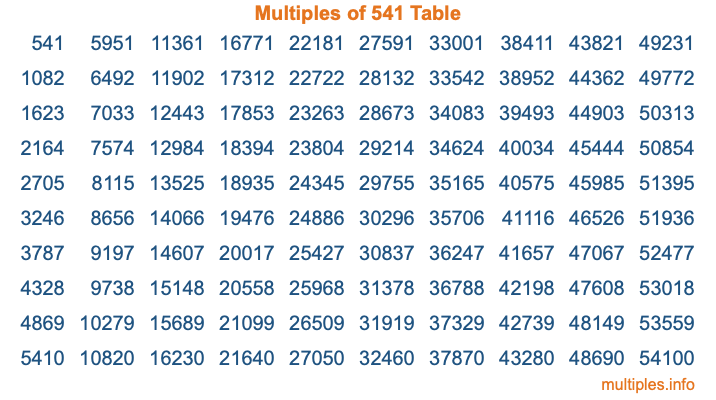Multiples of 541Welcome to the Multiples of 541 page. Here we will first teach you everything you will ever need to know about the multiples of 541, and then give you a study guide summary of everything we taught you to make sure you remember it all. Use this page to look up facts and learn information about the multiples of 541. This page will make you a multiples of five hundred forty-one expert!

Definition of Multiples of 541
Multiples of 541 are all the numbers that when divided by 541 equal an integer. Each of the multiples of 541 are called a multiple. A multiple of 541 is created by multiplying 541 by an integer.

Therefore, to create a list of multiples of 541, you start with 1 multiplied by 541, then 2 multiplied by 541, then 3 multiplied by 541, and so on for as long as you want. Thus, the list of the first five multiples of 541 is 541, 1082, 1623, 2164, and 2705. To see a larger list of multiples of 541, see the printable image of Multiples of 541 further down on this page. We also have a category where you can choose any nth multiple of 541.

Multiples of 541 Checker
The Multiples of 541 Checker below checks to see if any number of your choice is a multiple of 541. In other words, it checks to see if there is any number (integer) that when multiplied by 541 will equal your number. To do that, we divide your number by 541. If the the quotient is an integer, then your number is a multiple of 541.

Is  a multiple of 541?

Least Common Multiple of 541 and ...
A Least Common Multiple (LCM) is the lowest multiple that two or more numbers have in common. This is also called the smallest common multiple or lowest common multiple and is useful to know when you are adding our subtracting fractions. Enter one or more numbers below (541 is already entered) to find the LCM.

Check out our LCM Calculator if you need more details about the Least Common Multiple or if you need the LCM for different numbers for adding and subtraction fractions.

nth Multiple of 541
As we stated above, 541 is the first multiple of 541, 1082 is the second multiple of 541, 1623 is the third multiple of 541, and so on. Enter a number below to find the nth multiple of 541.

th multiple of 541

Multiples of 541 vs Factors of 541
541 is a multiple of 541 and a factor of 541, but that is where the similarities end. All postive multiples of 541 are 541 or greater than 541. All positive factors of 541 are 541 or less than 541.

Below is the beginning list of multiples of 541 and the factors of 541 so you can compare:

Multiples of 541: 541, 1082, 1623, 2164, 2705, etc.

Factors of 541: 1, 541

As you can see, the multiples of 541 are all the numbers that you can divide by 541 to get a whole number. The factors of 541, on the other hand, are all the whole numbers that you can multiply by another whole number to get 541.

It's also interesting to note that if a number (x) is a factor of 541, then 541 will also be a multiple of that number (x).

Multiples of 541 vs Divisors of 541
The divisors of 541 are all the integers that 541 can be divided by evenly. Below is a list of the divisors of 541.

Divisors of 541: 1, 541

The interesting thing to note here is that if you take any multiple of 541 and divide it by a divisor of 541, you will see that the quotient is an integer.

Multiples of 541 Table
Below is an image of the first 100 multiples of 541 in a table. The table is in chronological order, column by column. The first column has the first ten multiples of 541, the second column has the next ten multiples of 541, and so on.The Multiples of 541 Table is also referred to as the 541 Times Table or Times Table of 541. You are welcome to print out our table for your studies.

Negative Multiples of 541
Although not often discussed or needed in math, it is worth mentioning that you can make a list of negative multiples of 541 by multiplying 541 by -1, then by -2, then by -3, and so on, to get the following list of negative multiples of 541:

-541, -1082, -1623, -2164, -2705, etc.

Multiples of 541 Summary
Below is a summary of important Multiples of 541 facts that we have discussed on this page. To retain the knowledge on this page, we recommend that you read through the summary and explain to yourself or a study partner why they hold true.

There are an infinite number of multiples of 541.

A multiple of 541 divided by 541 will equal a whole number.

541 divided by a factor of 541 equals a divisor of 541.

The nth multiple of 541 is n times 541.

The largest factor of 541 is equal to the first positive multiple of 541.

541 is a multiple of every factor of 541.

541 is a multiple of 541.

A multiple of 541 divided by a divisor of 541 equals an integer.

541 divided by a divisor of 541 equals a factor of 541.

Any integer times 541 will equal a multiple of 541.

Multiples of a Number
Here you can get the multiples of another number, all with the same attention to detail as we did for multiples of 541 on this page.

Multiples of
Multiples of 542
Did you find our page about multiples of five hundred forty-one educational? Do you want more knowledge? Check out the multiples of the next number on our list!

Copyright  |   Privacy Policy  |   Disclaimer  |   Contact